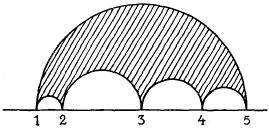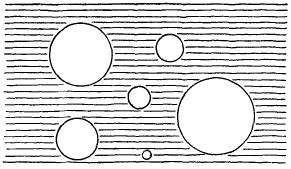## 7-2-52. Felix Klein to H. Poincaré, English translation

Leipzig, 2 July 1881

Dear Sir!

Allow me to answer at once the various questions you pose in your welcomed letter of 27 June.

1. In Volume 14 I described in detail the congruence groups $\alpha\equiv\delta\equiv 1,\ \beta\equiv\gamma\equiv 0\pmod{n}$ for $n=5$ (where by means of simultaneous deformation of the edges one obtains the icosahedron) and for $n=7$. The general case $n=$ a prime number is the topic of a memoir by Dyck, now in press.11endnote: 1 Dyck (1881). I have not yet completed the investigation of the case where $n$ is composite.

2. A “Geschlecht im Sinne der Analysis Situs” is associated with every closed surface. It is equal to the largest number of closed curves that one can draw on the surface without dividing it in two. If one now considers the surface in question as the image of the values of the numbers $w$, $z$ satisfying an algebraic equation $f(w,z)=0$, then its genus is that of the equation. Your genus and my Geschlecht are thus in fact the same number; only my interpretation is presumably more clearly associated with the Riemann surface and the definition of $p$ that it affords.

3. There do exist, however, in the group of modular functions, subgroups with asymmetric fundamental polygon, including in particular, as I have shown in Volume 14, those subgroups corresponding to the resolvents of the modular equation for $n=7$ and $n=11$.

4. It is of course well-known to me that in the case of a polygonthe circles should not intersect when extended towards the exterior if one wishes to form a single-valued function. In my opinion, one should concentrate precisely on this point in order to prove that the coordinates $w$, $z$ of the points of any algebraic curve can be represented by a single-valued function invariant under linear transformations. I will now indicate to you how far I have advanced with this question. By work of Schwarz and Weierstrass, one can always map the half-plane onto a polygon of circular arcs:in such a way that the points I, II, III, IV, V corresponding to the points 1, 2, 3, 4, 5 of the boundary of the half-plane are positioned arbitrarily. Suppose now that I, II, III, IV, V, …are the branch points of an algebraic function $w(z)$ and that this algebraic function has no other branch points. Then obviously $w$ and $z$ are single-valued functions of the desired sort of auxiliary variables in the plane of the indicated polygon. If, therefore, all of the branch points of an algebraic function $w(z)$ lie on a circle in the $z$-plane, then the answer to the question is immediately in the affirmative. What if, however, that is not the case? Then I arrive, in fact, at polygonsof the type noted last time. If the figure has no symmetry, I obtain (by establishing associated differential equations of the form $\frac{\eta^{\prime\prime\prime}}{\eta^{\prime}}-\frac{3}{2}\left(\frac{\eta^{% \prime\prime}}{\eta^{\prime}}\right)^{2}=R(z)$, which I have dealt with before) a fundamental region in similar fashion, where the edges meet tangentially, and which, moreover, are grouped together in pairs by means of certain linear substitutions. But I cannot prove that this fundamental region together with its iterates covers only a part of the complex plane. And this difficulty has held me up for a long time.5. Furthermore, one obtains other remarkable examples of discontinuous groups if one takes an arbitrary number of pairwise disjoint circles and reflects them onto one another by inversions. For greater clarity I have shaded the part of the plane exterior to all the circles, representing the fundamental half-polygon. These groups have been investigated occasionally by Schottky (Journal de Borchardt, Volume 83, pp. 300–351) without bringing their fundamental significance to light.22endnote: 2 Schottky (1877).

6. Riemann’s principles don’t at first yield any means for actually constructing a function whose existence has been established. One is therefore inclined to consider them as uncertain even though the results following from them are correct. On the other hand, in connection with the abovementioned mapping of a polygon of circular arcs, Weierstrass and Schwarz have effectively determined the relevant constants by means of convergent processes. If one is prepared to use Riemann’s principles, then one can prove the following very general theorem. Suppose given a polygon with one or more separate boundary components. The polygon may have several sheets, joined at branch points. Each boundary component is to be made up of several pieces, each of which can be transformed into another via a prescribed linear substitution. One can then always construct a function with arbitrarily prescribed discontinuities in the interior of the polygon and with real part taking on certain prescribed periodicity moduli as one passes from one piece of the boundary to the corresponding one by traversing the interior of the polygon. These functions include, in particular, those that are single-valued everywhere in the interior of the polygon and take the same value at each pair of corresponding points of the boundary. The proof is along precisely the same lines as that given by Riemann in §12 of the first part of his “Abelian Functions” [Rie1857] in the case of the special polygon made up of $p$ parallelograms arranged one above the other and joined by means of $2p-2$ branch points. This theorem, which by the way I have only fully established in the last few days, includes, it seems to me, all the existence proofs you mention in your notes as special cases or easy inferences. Incidentally, my theorem, like many that I write down these days, is not yet formulated precisely; otherwise I would have had to go into much more detail; you will easily get my meaning.

7. Allow me to make a remark concerning another of your publications [Poin1881a]. You say that the $\theta$-functions resulting from inversion of algebraic integrals on curves of genus $p$ are not $\theta$-functions of the most general sort. You could not know that in Germany precisely these ideas are common knowledge: a large number of young mathematicians are engaged in finding the conditions distinguishing so-called Riemann $\theta$-functions from general $\theta$-functions. On the other hand, I am surprised that you give the number of moduli of Riemann $\theta$-functions as $4p+2$, whereas in fact it should be $3p-3$. Haven’t you read the relevant explanations of Riemann? And aren’t you aware of the whole discussion that Brill and Noether settled in Volume 7 of Math. Annalen, pp. 300–307?

Hoping to hear from you soon, very respectfully yours,

F. Klein

PTrL. Slightly emended from the translation by R. Burns of the original German (§ 4-47-7) in Saint-Gervais (2016). See also the French translation (§ 7-2-29).

Time-stamp: "20.09.2023 18:41"

## References

• W. Dyck (1881) Versuch einer übersichtlichen Darstellung der Riemann’schen Fläche, welche der Galois’schen Resolvente der Modulargleichung für Primzahltransformation der elliptischen Functionen entspricht. Mathematische Annalen 18, pp. 507–527. Cited by: endnote 1.
• H. P. d. Saint-Gervais (2016) Uniformization of Riemann Surfaces: Revisiting a Hundred-Year-Old Theorem. European Mathematical Society, Zurich.
• F. Schottky (1877) Ueber die conforme Abbildung mehrfach zusammenhängender ebener Flächen. Journal für die reine und angewandte Mathematik 83, pp. 300–351. Cited by: endnote 2.